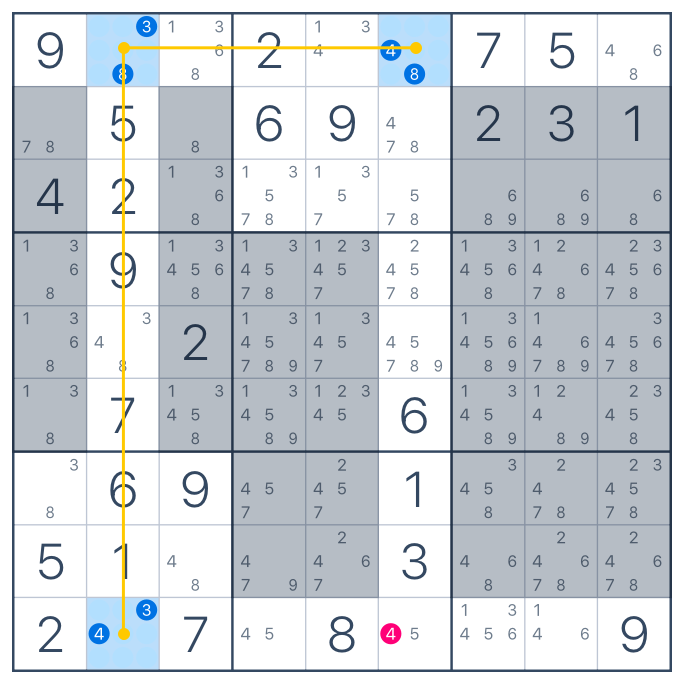# "Y-wing" technique.

"Y-Wing" technique is similar to "X-Wing", but it based on three corners instead of four.

Let's take a look at this technique with an example.

To start, we need to find a cell with exactly two notes. We'll call this cell a pivot.
Then, we'll look for two more cells with 2 notes as well. These cells (called pincers) should be in the same row, column or block as the pivot. One of the two numbers in each pincer should be the same as in the pivot. The other number is the same for both pincers.Now let's look where the both pincers intersect. That would be a cell in the bottom row. If that cell contains a note that is shared by both pincers, we can eliminate it. In this case it's number 4, because there's 4 in both pincers.That is how "Y-wing" technique works. This is an advanced sudoku strategy. It may take you some time and practice to figure it out.